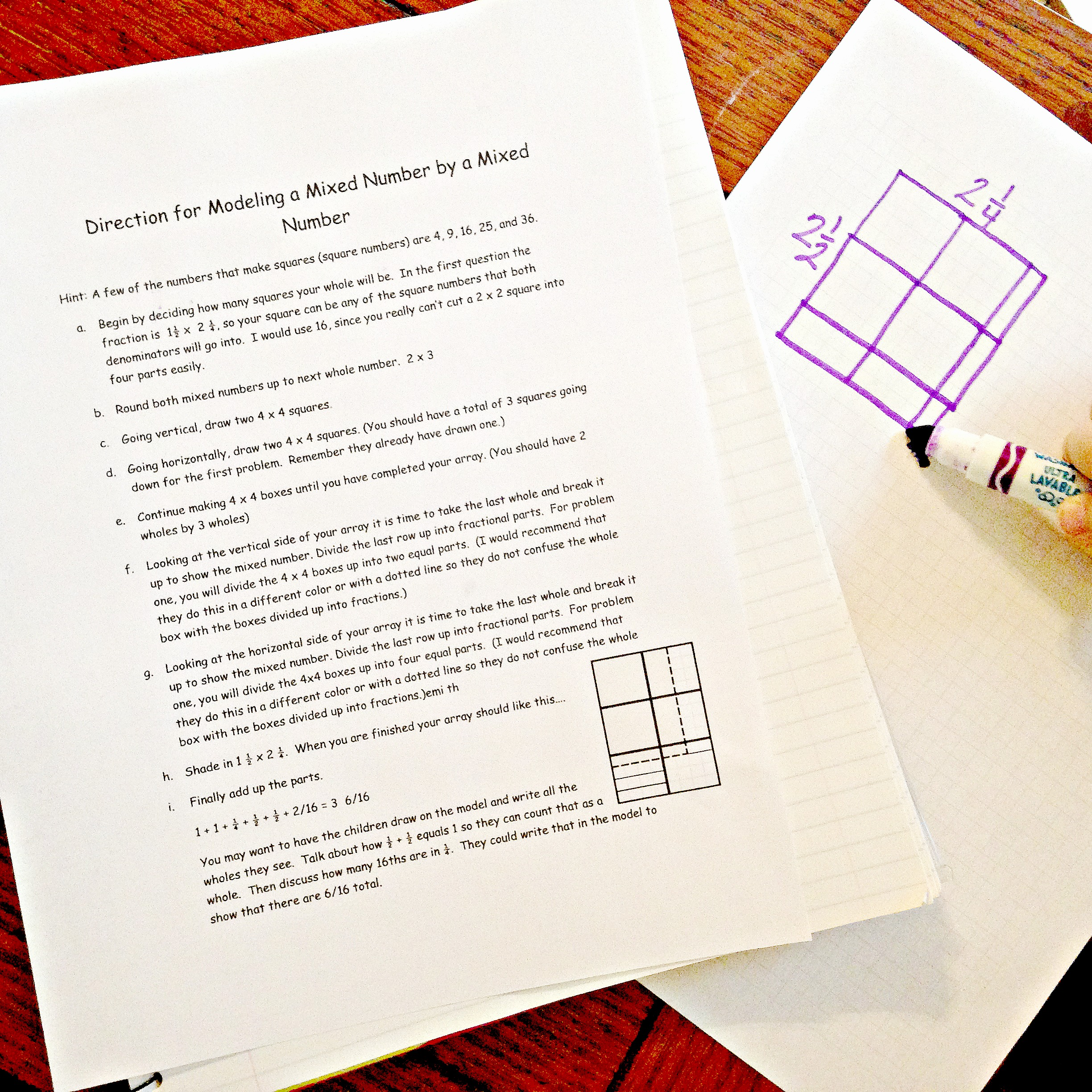# Multiplying Mixed Numbers | Free Printable

## Multiplying Mixed Numbers

Grab a free step-by-step directions for multiplying mixed numbers with a visual model. It includes practice problems for the children too.

One of the most common math operations that students learn in school is multiplying mixed numbers. This can be a bit confusing at first, but with a little practice, it becomes just another math skill.

*This post contains Amazon affiliate links

This lesson comes with a visual teaching component. I love drawing in math. When my children can visualize what they are calculating, I feel very happy. And I definitely love it when complex problems become easier for my kids.

So, how do you do this when teaching something like multiplying mixed numbers by mixed numbers?

## Multiplying Mixed Fractions using Base Ten Blocks

For this multiplying fractions visual, I would begin by getting your trusty base ten blocks out and have your students build an array!

Let’s say that you want your children to model what 1 1/10 x 1 3/10 would look like in an array. They can do this in just a few simple steps.

1. Before you begin, remind your eager children that an array must always be a rectangle, or if you can get them to tell you that, even better! Also, let them know that the flat will equal one whole today.

Discuss what the long equals and the cube equals. They should be able to answer this by knowing that if the flat equals one than a long is 1/10 and a cube 1/100.

2. Now it is time for the fun part, begin building the array. Start with 1 1/10 and build it vertically. I’m all for asking questions instead of telling.

“How many flats do I need?”

“How many cubes?”

Direct the students to place one flat and one long vertically on their desks.

3. Then move on to 1 3/10. Discuss that this fraction will be horizontal. Again, ask the same questions though you might add a twist.

How many flats do I need to add to my area? The answer is none. You already have one whole going horizontally.

You do not need to add another. All that needs to be added is 3/10.

4. Finally, ask your brilliant children what is wrong with the array. If you have spent enough time with arrays, they should recognize that it is not a rectangle.

Again, ask what could be done to fix it. I’m sure that someone will come up with the idea to add cubes to finish up the array.

## How to Multiply Mixed Numbers Using Arrays

Now that the children have a complete square or rectangle,  it is time to add up the base ten blocks.

1 + 3/10 + 1/10 + 3/100 = 1  43/100

I would like to add that I did all this in my head. I added the pictures later and double checked myself. Years ago if you had asked me to do 1  1/10 x 1  3/10 in my head I would have laughed at you. Now, visualizing the model has allowed me to be able to figure this out without writing a single thing down.

Depending on the needs of your students, you can do a few more using the base ten blocks or you can move on to the multiplying mixed numbers worksheet below.

## How to Multiply Mixed Fractions: Step-by-Step Directions

The freebie that is provided at the end of the post, will take you and your students step-by-step through drawing an array in a math journal.

I highly recommend that each student have a journal with grid paper. It is imperative that they use the grids to help them draw and then figure out any problem presented to them.

They also need colored pencils so that they can shade each part a different color. This helps them when it comes to adding up the array.

## Multiplying Mixed Fractions Word Problems

After the students have finished drawing an array, it is time for some practice.

1. First, there are a few word problems for the students to solve. They are required to draw a model, create two expressions, and then solve using the algorithm.

2. Next, students are given models to explore. The students need to figure out the equation that each model equals.

Building arrays can be a lot of fun! They are extremely helpful to our students who need to visualize math and are intriguing to the artist sitting in our classes. Enjoy exploring these arrays!!!

## Multiplying Mixed Numbers Worksheet

The free worksheet below will help your children or students practice how to multiply mixed fractions. If your students need more help with mixed numbers check out Subtracting Mixed Numbers While Regrouping (Common Denominators).

nfandnfmodelmultiplicationofamixednumberbyamixednumber